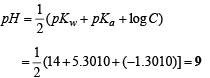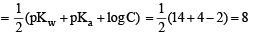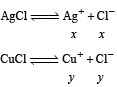Courses

# Integer Answer Type Questions: Equilibrium | JEE Advanced Notes | EduRev

## JEE : Integer Answer Type Questions: Equilibrium | JEE Advanced Notes | EduRev

The document Integer Answer Type Questions: Equilibrium | JEE Advanced Notes | EduRev is a part of the JEE Course Class 11 Chemistry 35 Years JEE Mains &Advance Past yr Paper.
All you need of JEE at this link: JEE

Q. 1. 0.1 M NaOH is titrated with 0.1 M HA till the end point; Ka for HA is 5.6 × 10–6 and degree of hydrolysis is less compared to 1. Calculate pH of the resulting solution at the end point.

Ans. 9

Solution. HA + NaOH → NaA + H 2O

At the end point, the solution contains only NaA whose concentration is 0.1/2 = 0.05 M

Since the salt NaA is formed by strong alkali (NaOH) and weak acid HA (indicated by its low Ka value), its pH can be evaluated by the following relation.Q. 2. The dissociation constant of a substituted benzoic acid at 25°C is 1.0 × 10–4. The pH of a 0.01 M solution of its sodium salt is

Ans. 8

Solution. pH of sodium salt of weak acidQ. 3. Amongst the following, the total number of compounds whose aqueous solution turns red litmus paper blue is

KCN, K2SO4, (NH4)2C2O4, NaCl, Zn(NO3)2, FeCl3, K2CO3, NH4NO3 and LiCN

Ans. 3

Solution. KCN, K2CO3 and LiCN are the salts of weak acid and strong base. So, their aqueous solutions turns red litmus paper blue.

Q. 4. The total number of diprotic acids among the following is:

H3PO4, H2SO4, H3PO3, H2CO3, H2S2O7, H3BO3, H3PO2, H2CrO4 and H2SO3.

Ans. 6

Solution. Diprotic acids are H2SO4, H3PO3, H2CO3, H2S2O7, H2CrO4 and H2SO3.

Q. 5. In 1 L saturated solution of AgCl [Ksp(AgCl) = 1.6 x 10–10], 0.1 mol of CuCl [Ksp(CuCl) = 1.0 x 10–6] is added. The resultant concentration of Ag+ in the solution is 1.6 x 10–x.

The value of “x” is

Ans. 7

Solution. Let the solubility of AgCl is x mol litre-1 and that of CuCl is y mol litre-1∴ Ksp of  AgCl =  [Ag+] [Cl]

1.6 × 10–10 = x (x + y) ....(i)

Similarly,  Ksp of CuCl = [Cu+][Cl]

1.6 × 10–6 = y(x + y) … (ii)

On solving, (i) and (ii)

[Ag+] = 1.6 × 10–7 ∴ x = 7

Offer running on EduRev: Apply code STAYHOME200 to get INR 200 off on our premium plan EduRev Infinity!

54 docs|30 tests

,

,

,

,

,

,

,

,

,

,

,

,

,

,

,

,

,

,

,

,

,

;